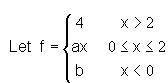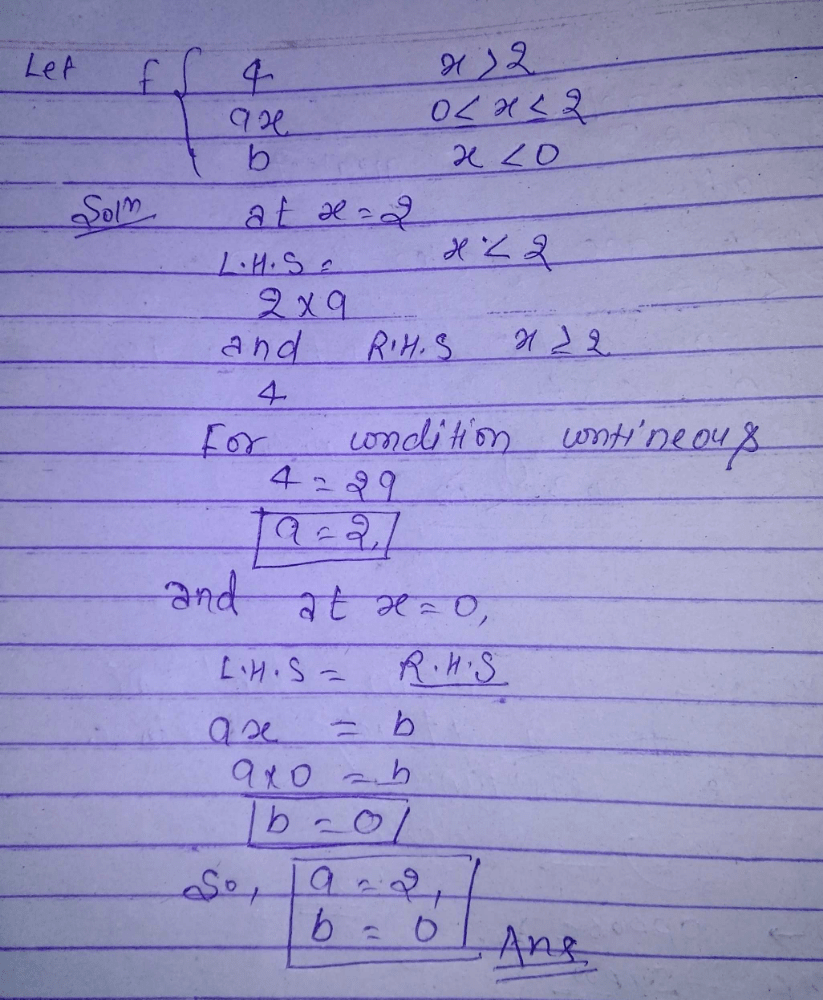Courses

#For what values of a and b, f is a continuous function.a)a=2,b=0b)a=1,b=0c)a=0,b=2d)a=0,b=0Correct answer is 'A'. Can you explain this answer? Related Test: Test: Continuity

## JEE Question

By Jayadev Subash · 2 weeks ago ·JEE
Bibhim Raj answered Nov 11, 2018Rakesh Pathak answered May 26, 2018
For continuity- LHL=RHL continuity at x=2 2a=4 a=2 continuity at x=0 b=0a b=0 Ok